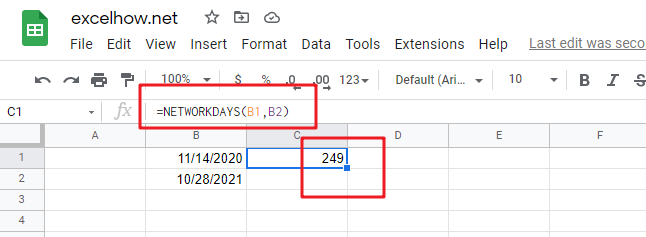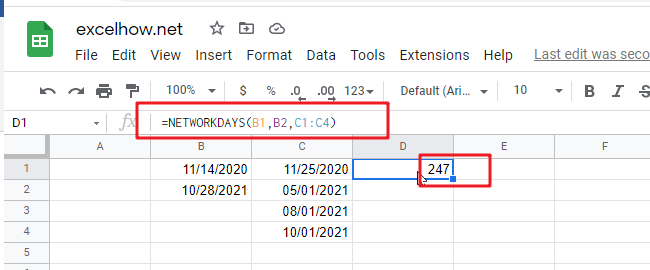# ExcelHow

This post will guide you how to use Google Sheets NETWORKDAYS function with syntax and examples.

## Description

The Google Sheets NETWORKDAYS function returns the number of net working days between tow given days.

The NETWORKDAYS function can be used to calculate the number of working days between dates in google sheets. And NETWORKDAYS will exclude weekends automatically. The purpose of this function is to get the number of working days between two dates and its returned values is a number that representing days.

The NETWORKDAYS function is a build-in function in Google Sheets and it is categorized as a Date function.

## Syntax

The syntax of the NETWORKDAYS function is as below:

=NETWORKDAYS (start_date, end_date, [holidays])

Where the NETWORKDAYS function argument is:

• Start_date -This is a required argument. The starting of date from which to calculate the number of working days.
• End_date – this is a required argument. The ending of date from which to calculate the number of working days.
• Holidays – this is an optional argument. A list of non-working days.

Note:

• NETWORKDAYS function only calculate all workdays, ignoring any time values.
• NETWORKDAYS function will exclude all Saturday and Sunday automatically.
• NETWORKDAYS function must be include both the starting date and ending date when calculating workdays.
• `Holidays` argument must be a date serial number values, or a date value returned by N function, or returned by DATE, DATEVALUE functions. It should be standard date values or date serial numbers.
• If you wish to use other days of week as the weekend, you can use the NETWORKDAYS.INTL function.

## Google Sheets NETWORKDAYS Function Examples

If you want to calculate the number of workdays between two dates in google sheets, you can use a formula based on the NETWORKDAYS function. And it will exclude all weekend days between the start dates and end dates. You need to specify two dates contain start date and end date so that you can get the number of workdays.

For example, you have two dates in range B1:B2, and you want to get the workdays between these two dates, you just need to use the following formula:

`=NETWORKDAYS(B1,B2)`

Type this formula in a blank Cell, and press Enter key in your keyboard.The above formula contains the NETWORKDAYS function but does not take into account holidays, if you wish to take into account holidays( a range in C1:C4), just using the following formula:

`=NETWORKDAYS(B1,B2,C1:C4)`